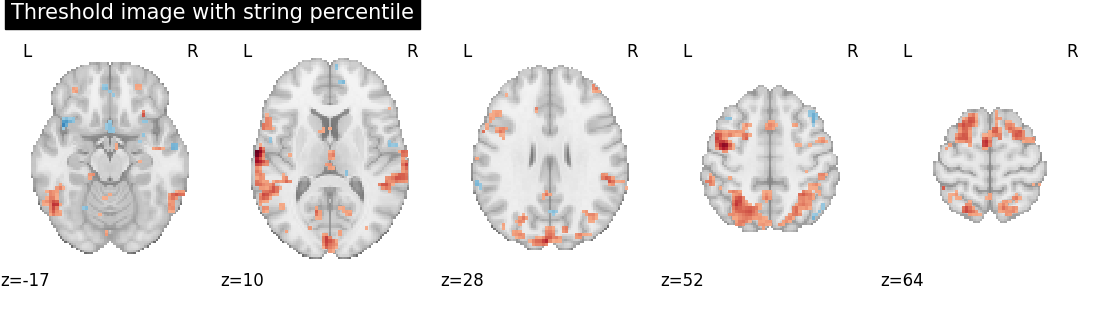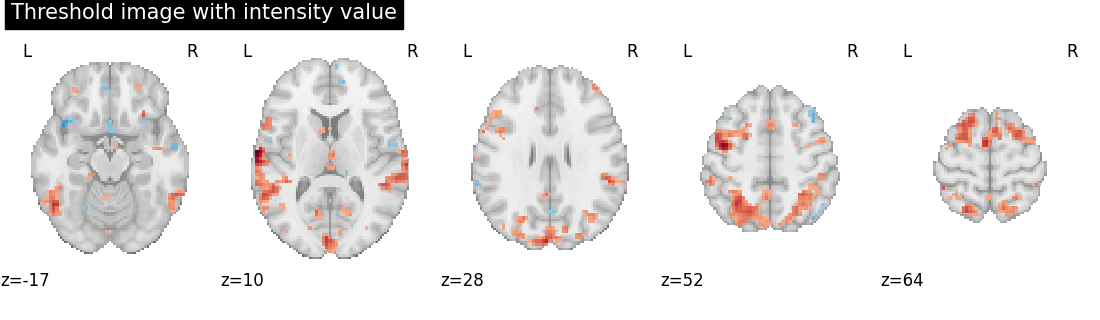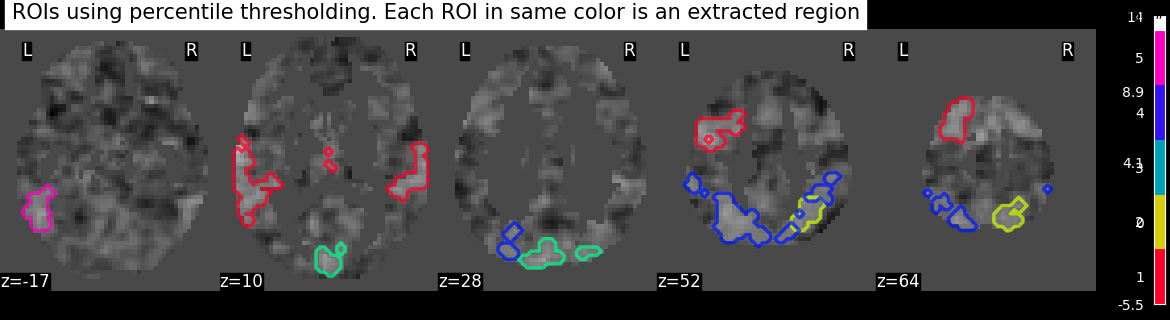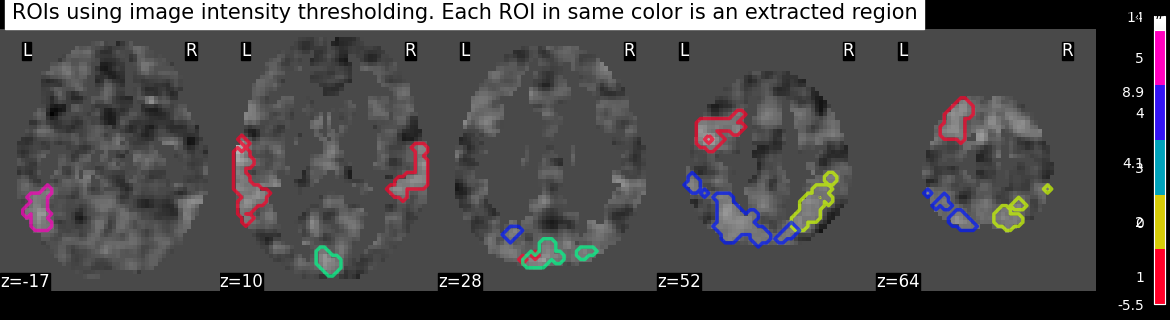# Region Extraction using a t-statistical map (3D)#

This example shows how to extract regions or separate the regions from a statistical map.

We use localizer t-statistic maps from `nilearn.datasets.fetch_neurovault_auditory_computation_task` as an input image.

The idea is to threshold an image to get foreground objects using a function `nilearn.image.threshold_img` and extract objects using a function `nilearn.regions.connected_regions`.

```from nilearn import datasets

tmap_filename = localizer.images
```

Threshold the t-statistic image by importing threshold function

```from nilearn.image import threshold_img

# Two types of strategies can be used from this threshold function
# Type 1: strategy used will be based on scoreatpercentile
threshold_percentile_img = threshold_img(
tmap_filename, threshold="97%", copy=False
)

# Type 2: threshold strategy used will be based on image intensity
# Here, threshold value should be within the limits i.e. less than max value.
threshold_value_img = threshold_img(tmap_filename, threshold=3.0, copy=False)
```

Visualization Showing thresholding results by importing plotting modules and its utilities

```from nilearn import plotting

# Showing percentile threshold image
plotting.plot_stat_map(
threshold_percentile_img,
display_mode="z",
cut_coords=5,
title="Threshold image with string percentile",
colorbar=False,
)

# Showing intensity threshold image
plotting.plot_stat_map(
threshold_value_img,
display_mode="z",
cut_coords=5,
title="Threshold image with intensity value",
colorbar=False,
)
```
••```<nilearn.plotting.displays._slicers.ZSlicer object at 0x7ff9da433fd0>
```

Extracting the regions by importing connected regions function

```from nilearn.regions import connected_regions

regions_percentile_img, index = connected_regions(
threshold_percentile_img, min_region_size=1500
)

regions_value_img, index = connected_regions(
threshold_value_img, min_region_size=1500
)
```

Visualizing region extraction results

```images = [regions_percentile_img, regions_value_img]
for image, strategy in zip(images, ["percentile", "image intensity"]):
title = (
f"ROIs using {strategy} thresholding. "
"Each ROI in same color is an extracted region"
)
plotting.plot_prob_atlas(
image,
bg_img=tmap_filename,
view_type="contours",
display_mode="z",
cut_coords=5,
title=title,
)
plotting.show()
```
••Total running time of the script: (0 minutes 10.167 seconds)

Estimated memory usage: 9 MB

Gallery generated by Sphinx-Gallery